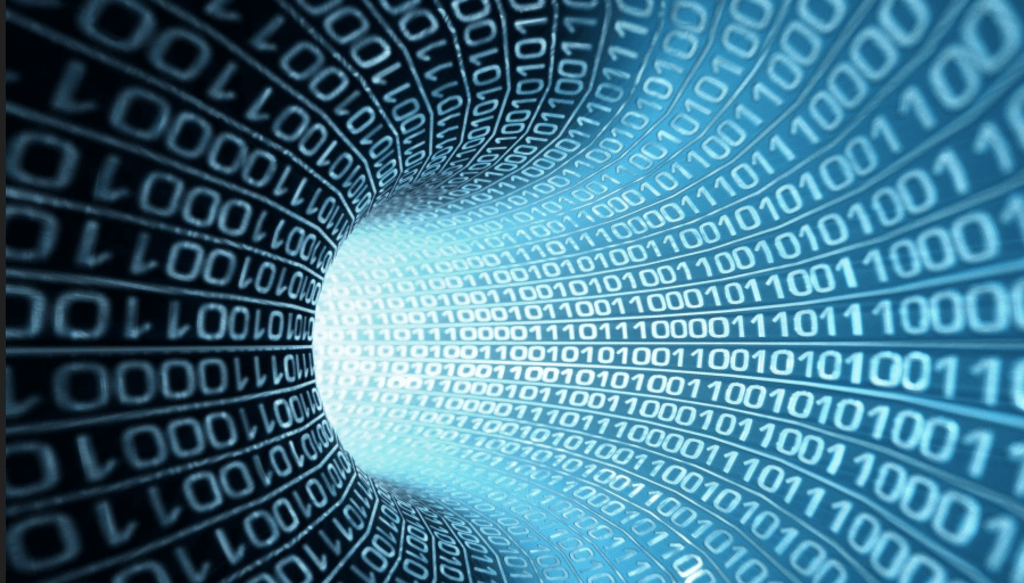# Everything You Need to Know About Decimal and Binary Numbers

by Aaron Smith 7 months ago in how to

### Get to know about the binary and the decimal numbers. The differences and where these numbers are used.Decimal numbers are the simplest numbering system as it consists of just ten numbers. These numbers include from 0 to 9. The decimal numbering system is widely known as the base 10 numbers. Decimal can also be identified for containing separator, it has the decimal numbers on both left and the right side of the point. This number has one of the most important roles in the expansion of mathematics.

Binary numbers consist of just zeros and ones. These numbers are not used in our daily life, all the electronic devices, especially computers, use these numbers because computers only understand binary. Humans do not use binary in their daily routine life but all the commands given by them are converted into the binary and then processed for further operations.

As decimal numbers are the most common numbers, for that reason it has several advantages:

• It is really easy to encode and decode these numbers, we can also use this system in our daily routine life
• Most humans use these numbers for the mathematical operations
• They are very easy to understand and memorize

• Binary numbers consist of just zeros and ones. So, these numbers are less complex compared to other numbers like a decimal
• Binary number occupies the least amount of space in a system, for that reason, these numbers can easily be processed
• For security reasons, many software programmers use the binary number to store the password strings. This technique improves the security in a computer system

Difference between these two numbers

As discussed earlier, decimal numbers contain from zero to nine and binary contains zeros and ones. When it comes to a computer or an electronic system, it converts the decimal to binary and then processes the given number. For example, if you are entering a decimal number “44” then the computer will convert “44” into the binary. Well, you do not see the binary in the output, right? It converts back binary to decimal when it shows the answer to the users.

Where these numbers are used?

In most of the processing, binary numbers are used in the electronic or computing processors. Humans can easily understand decimal numbers where computers can read-only binary numbers. So, in many systems on the front-end, programmers use text or the decimal numbers, where on the back end they use binary numbers.

For example, many programmers use binary to store the data. They convert the given string or number of passwords into the binary value and then store that binary into the database for security reasons. This way of storing data has been very effective over the years. There are several packages also available to convert the string in the least amount of time.

Along with that, binary numbers are also used in the IOT systems, registers, computing properties, and the AI (Neural network) concept. So, these are some parts where the binary is used over the years.

Bottom line

Above, we have described the details about binary and decimal numbers. If you have any suggestions, then do let us know by dropping a comment or contacting us. We would be happy to hear from you and help you to solve your queries.

how toAaron Smith
Read next: Wearables vs The Virus | João Bocas | Engati Engage
Aaron Smith
See all posts by Aaron Smith